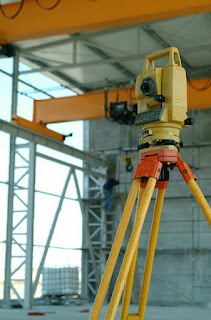WHAT IS THE TOTAL STATION AND WHAT USE IN SURVEYING AND MINING

# WHAT IS THE TOTAL STATION AND WHAT USE IN SURVEYING AND MINING

upendra kumar

TOTAL STATION

Total Station is an electronic or optical instrument for measure angle, distance and coordinates. It is a combination of transit theodolite with electronic distance meter.TOTAL STATION

Accessories of total station

a. Tribrach
b. Optical plummets.
c. Prism or reflectors.Prism reflector

The setting of the total station over a station

The setting of total station similar to theodolite.
A. Centring over a station.
B. Levelling of the instrument.
C. Elimination of parallax.

In the total station has an arrangement for digital display for centring and levelling also.

The total station takes the reading two type

By use of reflector.

In the total station taking a reading for survey, the purpose has two types.
1. By Angle, bearing and distance method it is used for traversing purpose.
2. By the coordinate method, It is used for all type of survey work. Most suitable survey method in this setting is a minimum of two coordinate of survey points required.
But in the total station has the arrangement for one point coordinate survey method also.
In the one point, coordinate method the total station is set up of known coordinate survey point the second point of coordinate known by the use of bearing and distance of other survey point or line making between first survey point and second survey point.

In the two coordinate survey points method is generally used in surveying.
In this method, the instrument is set on the first point whose coordinate is entered as an instrument station.
Second survey point where coordinate is also known is called back sight-reading. It is read by a reflector kept stand on second survey point.
Resection method. In this method the instrument is set anywhere point whose coordinate required, and reflector kept stand alternate on the survey points.

Reflectorless method.
In this method, the reading took a direct target to the object. Object should be still position and surface should be refraction in nature.
It is also used for remote elevation.

• Averaging multiple angle and distance measurements
• Correcting electronically measured distances from prism constant, atmospheric pressure, and temperature.
• Making curvature and refraction corrections to elevations determine by trigonometric levelling.
• Computing coordinates of survey points from horizontal angle and horizontal distance.

Use of the total station
Total station is the use of all type of survey work.
In the mining total station is used both types of mine underground and open cast.
a. Routine work of survey
b locate the borehole, outcrop, bench monitoring, system, blast control and quantity measurement.
c. It is also where GPS' is not worked properly.

Finally, give the data an x.y.z or NORTH,  EAST, ELEVATION
form.